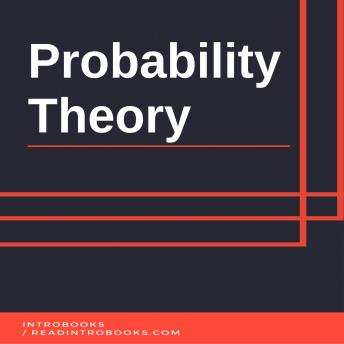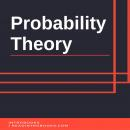# Probability Theory

#### Summary:

Analysis theory of any random phenomena is known as probability. Main resource for concept of probability is probability theory. Probability theory is said to be one of many most important concepts or branches of mathematics. Following objects are known as main concepts or central objects:

Random variablesStochastic processes Random events Measured quantitiesEvents are a kind of abstraction achieved through mathematical process. This abstraction is done on the base of non-deterministic events. Measured quantities can also be used for such abstraction. Measured quantities can be single occurrence or also follow random fashion for time evolving multiple occurrences. Making predictions about the outcome of random events is not always easy or even impossible sometimes. However, if influences are made on sequence of individual events, they will tend to follow certain patterns that are much easy to study, practice and predict. For instance, if outcome of coin flipping or roll of dice is influenced by outer factors such as friction, they will follow a specific pattern when proving outcome. Once this certain pattern is figured out and understood properly, it becomes much easier to predict the outcome with maximum accuracy. It means that it is hard to understand future outcome of subsequent random events that makes it hard to predict them. However, it is easy to predict pattern exhibited by these group of events that makes it easy to predict them and their corresponding events also. To describe such patterns certain mathematical results or concepts are used. Law of large numbers and central limit theorem are two main concepts that are used to study and describe such patterns followed by sequential random events.

Mathematical foundations of statistics consider probability theory as a practical part for any human activity in which, quantitative analysis of large data sets are involved. Involving in daily human activities makes probability theory a main concept of mathematics and statistics. It is also heavily involved in many mathematical and statistical concepts, which also makes it important for one to learn and understand basic concepts of this theory. Probability theory can also be used to describe complex systems, systems that hold only partial knowledge of their corresponding state. These descriptions are done the same way they are done in statistical mechanics. This is the main reason for which this theory is considered as a part of both mathematics and statistics. However, mathematics and statistics are parent theories for each other so any concept of mathematics can be a concept of statistics and any concept of statistics can be a concept of mathematics. Discovery of probabilistic behavior of physical phenomena is considered a great discovery in the field of physics. This probabilistic behavior was observed at atomic scales. This whole observation was heavily related to the concepts of probability theory. However, probability theory was not used as a complete base in that theory. This concept and research is broadly described in theory of quantum mechanics. Quantum mechanics is arguably the only field in physics, which is related with the concepts of probability theory. However, researches are still going on and more connections are much likely to be found out soon thanks to the deep relations between mathematics and physics.

## Probability Theory

#### by Introbooks##### Enter your email below to be notified as soon as it is available!

By clicking "Notify Me" you consent to receiving electronic marketing communications from Audiobooks.com. You will be able to unsubscribe at any time.